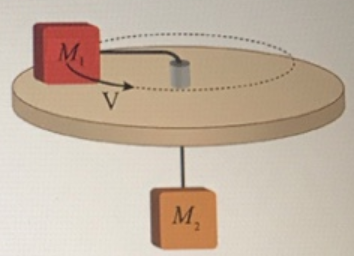# Problem: Two blocks, which can be modeled as point masses, are connected by a massless string which passes through a hole in a frictionless table. A tube extends out of the hole in the table so that the portion of the string between the hole and MI remains parallel to the top of the table. The blocks have masses M1 = 1.2 kg and M2 = 2.7kg.  Block 1 is a distance r = 0.95 m from the center of the frictionless surface. Block 2 hangs vertically underneath.Part (a) Assume that block two M2 does not move relative to the table and that block one, M1 is rotating around the table. What is the speed of block one, M1, in meters per second?Part (b) How much time, in seconds, does it take for block one, M1, to make one revolution?

###### FREE Expert Solution

Part (a)

Newton's second law:

$\overline{){\mathbf{\Sigma }}{\mathbf{F}}{\mathbf{=}}{\mathbf{m}}{\mathbf{a}}}$

Tangential acceleration in uniform circular motion:

$\overline{){\mathbf{a}}{\mathbf{=}}\frac{{\mathbf{v}}^{\mathbf{2}}}{\mathbf{r}}}$

M2g - T = 0

T = M1v2/r

80% (190 ratings)###### Problem Details

Two blocks, which can be modeled as point masses, are connected by a massless string which passes through a hole in a frictionless table. A tube extends out of the hole in the table so that the portion of the string between the hole and MI remains parallel to the top of the table. The blocks have masses M1 = 1.2 kg and M2 = 2.7kg.  Block 1 is a distance r = 0.95 m from the center of the frictionless surface. Block 2 hangs vertically underneath.Part (a) Assume that block two M2 does not move relative to the table and that block one, M1 is rotating around the table. What is the speed of block one, M1, in meters per second?

Part (b) How much time, in seconds, does it take for block one, M1, to make one revolution?